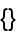#Function Repository Resource:

# CanonicalBranchPairs

Compute canonical branch pairs for a given multiway system

Contributed by: Jonathan Gorard
 ResourceFunction["CanonicalBranchPairs"][rules] generates a list of canonical (i.e. initial condition-independent) branch pairs for the multiway system with the specified rules. ResourceFunction["CanonicalBranchPairs"][rules,n] generates an association of resolved and unresolved canonical branch pairs for the multiway system with the specified rules after n steps.

## Details and Options

Rules can be specified in the following ways:
 {"lhs1"→"rhs1",…} string substitution system {{l11,l12,…}->{r11,r12,..},…} list substitution system [ rules ] cellular automaton system "type"→rules system of the specified type
Supported rule types include:
 "StringSubstitutionSystem" rules given as replacements on strings "ListSubstitutionSystem" rules given as replacements on lists "CellularAutomaton" rules given as a list of CellularAutomaton rule specifications "WolframModel" rules given as replacements on hypergraphs
ResourceFunction["CanonicalBranchPairs"] accepts both individual rules and lists of rules.
Options for ResourceFunction["CanonicalBranchPairs"] include:
 "GivePredecessors" whether to label branch pairs with their predecessor state "GiveResolvents" whether to label branch pairs with their resolvent state "IncludeSelfPairs" whether to include trivial branch pairs
In the theory of abstract rewrite systems, a critical pair (branch pair) is a pair of expressions that can be derived from a common expression by two different applications of a rewrite rule (i.e. either the same rule applied in two different places, or two different rules).
More generally, a branch pair indicates where a multiway evolution bifurcates.

## Examples

### Basic Examples (3)

Generate the list of all canonical branch pairs (i.e. critical pairs) for a string substitution system:

 In:=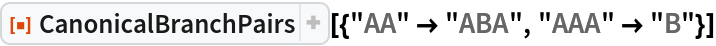Out=Generate the association showing which branch pairs converge after 2 steps and which do not:

 In:=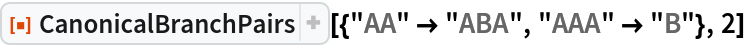Out=Show common predecessor states:

 In:=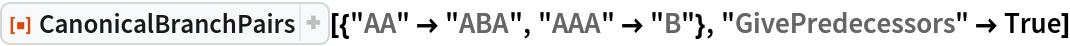Out=Show common resolvent states for resolved canonical branch pairs:

 In:=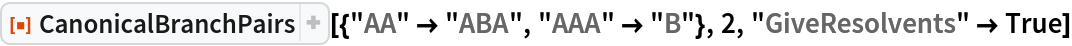Out=Show both common predecessors and common resolvents, where appropriate:

 In:=Out=CanonicalBranchPairs can handle Wolfram Models and other system types:

 In:=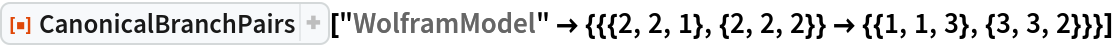Out=In:=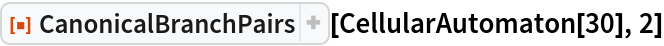Out=In:=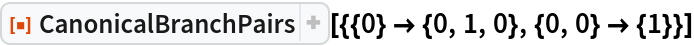Out=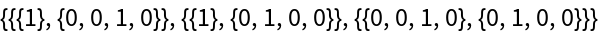### Scope (4)

#### System Types (4)

CanonicalBranchPairs supports both string and list substitution systems:

 In:=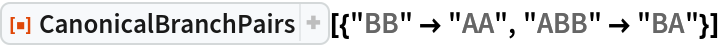Out=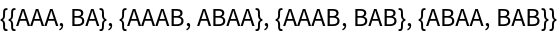In:=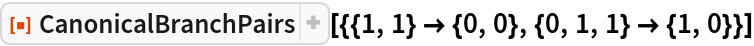Out=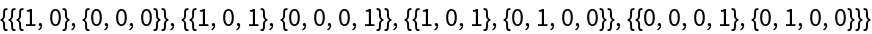CanonicalBranchPairs also supports multiway generalizations of cellular automata:

 In:=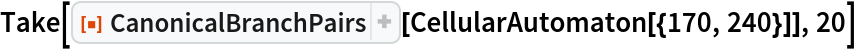Out=Generate all resolved branch pairs from left- and right- shift cellular automaton rules after 5 steps:

 In:=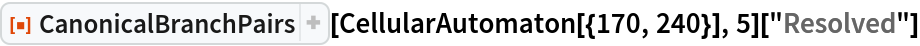Out=Determine that the rule 30 cellular automaton is not causal invariant:

 In:=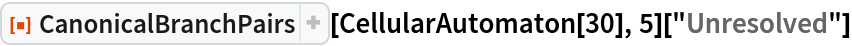Out=CanonicalBranchPairs also supports multiway generalizations of Wolfram Models:

 In:=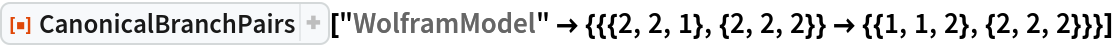Out=Determine that this Wolfram Model rule has unresolved branch pairs, and thus is not causal invariant:

 In:=Out=### Options (4)

#### Predecessors and Resolvents (2)

By default, CanonicalBranchPairs returns only a list of canonical branch pairs:

 In:=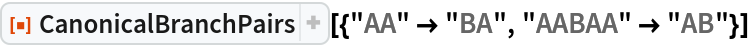Out=Common predecessor states can be shown using "GivePredecessors":

 In:=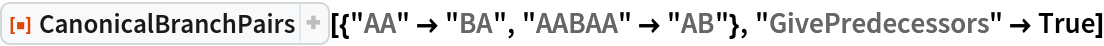Out=Similarly, CanonicalBranchPairs with a step count by default lists only resolved and unresolved canonical branch pairs:

 In:=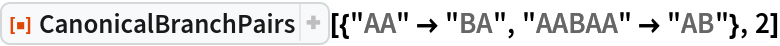Out=Common resolvents of resolved branch pairs can be shown using "GiveResolvents":

 In:=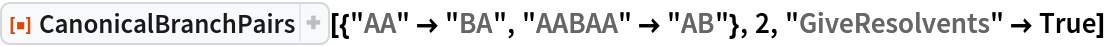Out=Show both common predecessors and common resolvents, where appropriate:

 In:=Out=#### Self Pairs (2)

By default, CanonicalBranchPairs does not include self pairs (i.e. trivial critical pairs):

 In:=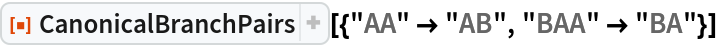Out=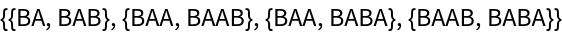Self pairs can be included using “IncludeSelfPairs”:

 In:=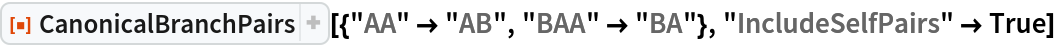Out=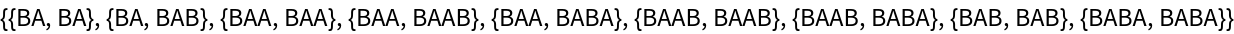### Applications (2)

#### Causal Invariant Rules (2)

Prove that the Xor and And functions are total causal invariant (due to associativity):

 In:=Out=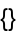In:=Out=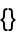On the other hand, the Nand function is not associative, so it is not total causal invariant:

 In:=Out=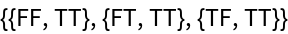### Properties and Relations (1)

CanonicalBranchPairs returns an empty list of unresolved canonical branch pairs if and only if the rule is total causal invariant:

 In:=Out=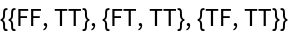In:=Out=